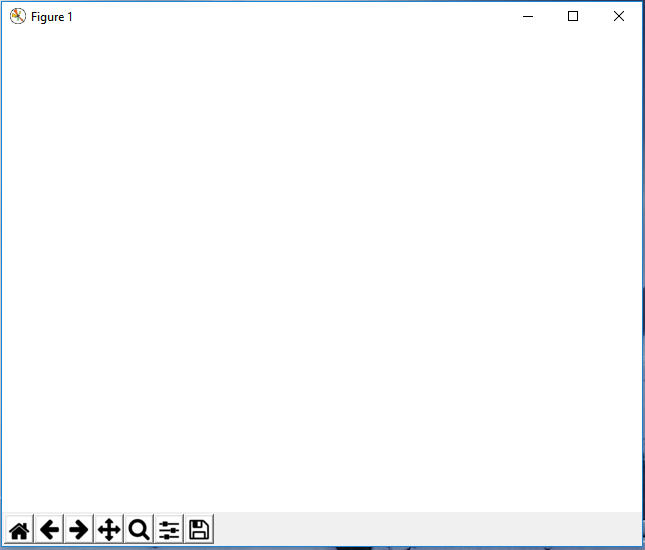﻿ How to Create an Empty Figure with Matplotlib in Python ﻿# How to Create an Empty Figure with Matplotlib in PythonIn this article, we show how to create an empty figure with matplotlib in Python.

So with matplotlib, the heart of it is to create a figure.

On this figure, you can populate it with all different types of data, including axes, a graph plot, a geometric shape, etc.

However, the first step really is to create a figure.

So how do we create an empty figure object with matplotlib?

This is shown in the following code below.

So the first thing we have to do is import matplotlib. We do this with the line, import matplotlib.pyplot as plt

We then create a variable fig, and set it equal to, plt.figure()

This creates a figure object, which of course is initially empty, because we haven't populated it with anything.

So we have a figure object stored in the variable, fig, that is empty.

To show this empty figure object, we use the line, fig.show()

This works if you're using a python IDE other than jupyter notebooks. If you are using jupyter notebooks, then you would not use, plt.show(). Instead you would specify in the code right after importing matplotlib, %matplotlib inline

This line allows the figure of a graph to be shown with jupyter notebooks.

After running the following code above, we get the following empty figure shown in the image below.Of course there is nothing in the figure, because we haven't written any code that populates the figure.

But we will do this in later tutorials.

And this is how to create an empty figure object with matplotlib with Python.

Related Resources

How to Randomly Select From or Shuffle a List in Python